## 4. 1. 7 Derivative Considerations

The discussed equations form a linear equation system whose solution vector gives an increment or update for the respective solution quantities. In the Newton scheme an equation system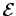is linearized in the neighborhood of a vector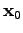of quantity values for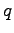.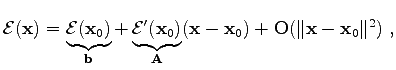(4.24)

where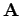denotes the system matrix and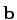denotes the right hand side vector. All terms of higher than second order are neglected. An extension of the linearized expressions might also contain second order expressions, however, the memory consumption is raised from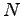to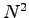in the worst case, whereis the number of unknown variables.

For the first order case, the evaluation of the residuum in the respective linearization vectorresults in the (inverse) right hand side. The evaluation of the derivatives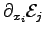in the linearization vectoryields the entries of the system matrix.

From this point of view, it can be stated that forming the derivatives from the discretized expression is performed implicitly when using linearized expressions. This is of course evident, when the Taylor expansion is considered, however, a lot of work for the differentiation of the respective equations can be saved. It also has to be stated that using this method for forming the derivatives only determines the derivative only for a given linearization vectorand not an analytic expression. This implies that such a method is not apt for the differentiation of general algebraic formulae, however, it performs well for the specific matter.

Michael 2008-01-16